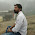# C++ Program For Linear Search

Problem:- C++ Program Linear Search or Linear search in C++ Program Example Code or Linear Search in C++ or C++ Program Linear Search in Array or Simple Linear Search Example Program in C++ or Linear Search In Array or Program to search an element in an array using Linear search or Linear Search Program in C, C++ or C++ program to do linear search in Array or C++ Program for Linear Search in Array or C++ Program to Implement Linear Search Algorithm or Linear search program in c++ using recursion or C++ Program: Implementation Of Linear Search or Program to Implement Linear Search in 1D array in C++ or Linear search program in c++ using recursion.

Logic:- Linear search is a simple search ie. line by line searching. For better understanding a linear search we are taking an example of an array and try to find out an element of an array. We compare element to each and every element of an array if the element matches with array elements then we print matches that element found or we can also print the index of an array. Now we Know that how Linear search worked, Now we are taking an example and performing a Linear Search on it.

Check This:- C Program For Linear Search

Explanation:- Let's take an example of an array suppose an array element is 10, 20, 30, 90, 80, 70, 40, 50, 60, 100. We can clearly see that the array size is 10 and an array index is started 0 to 9 and we have to find the element 50 now take a step by step action of our program and see how Linear search works.

Step 1:- First we compare the element 50 to the array first element then second, third and so on. We can clearly see that our elements 50 not matching with the array element 10, so increase an index of an array and compare with the second element then third until our matching element Not Matches with the array elements.

Step 2:- After the element matches with the array element than we print the Message or index that we have found the element, or if our element not matched with the array elements then we can print the message element not found.

Key Notes:- Here are some feature of a Linear search.

1. Linear search worked on Sorted and Unsorted Data.
2. Linear search Worst-case performance =====>O(n)
3. Linear search Best-case performance ======>O(1)
4. Linear Search Average performance =======>O(n)
5. Linear Search Worst-case space complexity =>O(1)

Check:- Geeksforgeeks solution for School, Basic, Easy, Medium, Hard in C++.

Solution:-

#include<iostream>
#include<stdlib.h>
using namespace std;
int main()
{
//C++ program for linear search

int *a,i,itm,s,flag=0;

cout<<"==================================";
cout<<"\nC++ Program For Linear Search\n";
cout<<"==================================\n";

cout<<"\nEnter The Size Of An Array :";

cin>>s;

a=(int*)malloc(s*sizeof(int));

cout<<"\nEnter An Elements Of An Array"<<endl;

for(i=0;i<s;i++)
{
cin>>a[i];
}

cout<<"\nEnter The Number You Want To Search In An Array :";
cin>>itm;

for(i=0;i<s;i++)
{
if(a[i]==itm)
{
flag=1;
}
}

if(flag==1)
{
cout<<"\nNumber Found In an Array \n";
}
else
{
}
}

Output:-

1.hi can i have the code with biary and linear together in one program

1.Hi, Thanks for connecting with US here is a link of Binary Search

2.thanks for this

1.Thanks for liking and support.